Next: Input Cards Up: usermanual Previous: Output Description   Contents

# COHERENCE

In this chapter the processing of step COHERENCE is described.

In this step the following is computed. The (complex) coherence image is computed, with or without subtraction of the reference phase and the radarcoded DEM phase. The reference phase is subtracted if there is a 2d-polynomial in the products result file (result of step FLATEARTH). It is not subtracted if this is not in the result file or if the number of coefficients is set to 0. For a proper estimation of the coherence map, especially over mountainous areas, the radarcoded DEM phase should also be subtracted. In this case, the result of step COMPREFDEM is required in the products result file. This is the default procedure since v4.01.

The complex coherence image between two images is defined as: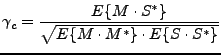(1)

Where: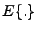is the expectation;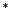is the complex conjugated;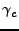is the complex coherence;
M is the complex master image;
S is the complex slave image (possibly minus (complex) reference phase and DEM phase: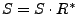).

The coherence is defined by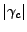and its estimator as: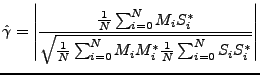(2)

Multilooking can be performed to reduce noise. Usually a ratio of (line:pixel) = 5:1 between the factors is chosen to obtain more or less square pixels (20x20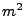for factors 5 and 1). (The resolution decreases of course if multilooking is applied.)

Data buffering is applied for the memory considerations.

SubsectionsNext: Input Cards Up: usermanual Previous: Output Description   Contents
Leijen 2009-04-14